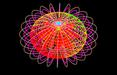HOMEAbout meCoursesLinksColumnGalleryPublicationsAMS JournalsMAA JournalsDe Gruyter JournalsarXiv.orgEJCSM: E-Journal

EJCSM: E-Journal of Cross Sections in MathematicsThe journal EJCSM is on construction but I invite readers to read articles I seldom  post  on this page and few of  them are available in arXiv.orgMy profile and URL address in  a global  network of  scientists  & researchers
Shortly I will have consecutive postings on:

1. Generalized Functions
2. Distributional/ weak  Derivatives
3. Function Spaces in particular Sobolev SpacesThis block/module will be for calculus lessons:

I will post rudiments of calculus in the following  order:

Sets-Functions
Limits-Continuity
Differentiation-Techniques of Differentiations-Applications of Differentiation in different  areas.

Antiderivative or Integration
Techniques of Integrations-Applications of Integrations.

Knowing all these concepts will give a student a good momentum  to learn mathematics   at universities  and colleges. Threfore stay tune for the fun.
Mathematically, aid is an initial condition, while growth is a behavior of a system which in most cases has nothing to do with initial conditions
- Dejenie A. Lakew, Ph.D.Definite Integral :

lim ∑_{k=0}ⁿ =∫_{a}^{b}
n→∞
E
J
C
S
M

" What science can there be more noble, more excellent, more useful for mankind,  more admirably high and demonstrative than mathematics " - Benjamin Franklin.SHINING & SPARKLING MATHEMATICAL IMAGES -- BY DEJENIE ALEMAYEHU LAKEW, PH.D.Mathematics - from the   foundations through its courses of developments, using rules of logic, abstractions and reason aided by  the imagination of the thinking friend of the human mind,   indeed is more  than any one of a common ordinary human languages. It is in fact  a supra-language of the greater universe    in which the human language oscillates as a barrier and element of imperfection in understanding the universe, communicating with it  and precisely behave accordingly and fit in the section that we are in. It is   the only universal supra-language that describes universal phenomena either that emanates from the human mind and imagination or that is observed from nature outside of the human mind in its every possible  aspects or coordinates of existence precisely with no ambiguity.  Mathematics though universal, but when used for special human purposes is also local. Locality here is not only in terms of validity, but in terms of   existence. The moon exists locally as a satellite of earth very close, but also exists in the universe as such      but with more properties ( who knows ) but with infinitely negligible ( small ) size.

Think for the moment why computers do perfectly with minimal possibility of errors, doing what we          intend them to do:  computers in flights, hospitals, banks, cars, etc. ? Because their language - the way they  receive input data,  process information  and  communicate their results to us is  through the only language   of no ambiguity -- Mathematics. Had it been one of the languages of man that computers use, I can imagine   how many tragedies have been committed from flights, hospitals, cars, etc, where computers are used,  since the advent of computers and their usage for man.

As a matter of  fact we sometimes say, the mathematics we write in human languages informal, while the      one we do in abstractions using  possible symbols of mathematics  formal. Here the formal descriptions of   the mathematics we do  are not bound to misinterpretations and thereby getting different results - which in   most cases are wrong results. But the formal ones always  precise, not open to distortions which when         happen reflected on the negative and un intended results. Almost all destructions that occurred through out  the history of humankind in dealing with each other and reading nature are due to misrepresentation,             misreading, misunderstanding, miscommunication and thereby getting un intended results and their                aftermath.
Then what better language of precision can somebody of a common sense imagine than Mathematics ?
~ Dejenie Alemayehu Lakew, Ph.D.∫_{[0,1]}exp(exp(x))dx = ∑_{k=0}^{∞}∑_{j=0}^{∞}((k^{j})/((k!)(j+1)!))
(d/(dx))∫_{a}^{x}ψ(t)dt = ψ(x)
∫_{a}^{b}ψ′(x)dx = ψ(b)-ψ(a)
∫udv = uv-∫vdu
(ψφ)′ = ψ′φ+ψφ′
Instant Calculus:
((ψ/φ))′ = ((ψ′φ-ψφ′)/(φ²))ℒ(C[a,b])=∫_{a}^{b}√(1+(ψ′(x))²)dx
φ′(ψ(φ))′=ψ′(φ)φ′Two Fundamental Theorems of CalculusFew of the basic rules of differentiationIntegration by parts
Length of an arc C: graph of  ψ on [a,b]∫_{a}^{b}udv = uv∣_{a}^{b}-∫_{a}^{b}vdu
ψ∣_{a}^{b}:=ψ(b)-ψ(a) - Function Evaluation
ψ:A→B, read as : ψ is a function from set A in to set B.  A- Domain of  ψ,  B- Co-domain of ψ
ψ[A]:={ψ(a):a∈A}- Range of ψ - Function denotation and associated sets.
V=∫_{[a,b]}π(ψ(x))²dxVolume of a solid of revolution of a plane domain enclosed by graph of ψ on [a,b]
CALCULUS -
GATEWAY TO MATHEMATICSMathematics Problems Corner: